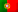Home > Technology > Computer Science > Programming > C++ > Singly linked lists in C++ (Part I)

# Singly linked lists in C++ (Part I)

Linked lists are data structures that consist in a set of nodes linked each other. There are different types of linked lists, such as: Singly linked lists, doubly linked lists and circular linked lists. In a similar way to dynamic arrays (see my articles “malloc and realloc functions in C / C++” and “Dynamic arrays with structs in C++“), linked lists allow us to insert many nodes as the computer’s memory allow, allocating memory as we need to insert new nodes. Let’s see some advantages and disadvantages of using lists against dynamic arrays:

• Inserting and removing nodes is easier and avoid the need of shifting data to the right or to the left inside an array.
• Implementing stacks and queues is easier with lists.
• They don’t need the implementation of a capacity controller and the usage of the realloc function.

• It is not possible to directly access a specific node of a list, being necessary a sequential reading of the nodes until we reach the desired node. Usually we only have a pointer to the head (beggining) of the list and, depending on the implementation, another pointer to the tail (end) of the list.
• Linked lists need to use more memory because, for each node (or element), we need to add a pointer to the next node (singly linked lists) or two pointers (doubly linked lists).
• Nodes are stored incontiguously in memory, thus increasing the access time to reach a specific node.

In this article, I’m going to speak about singly linked lists. For this kind of lists we need to have a pointer to the head and, for each node, a pointer to the next node.

The following picture illustrates a list of integer numbers: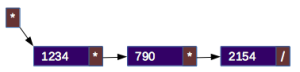The smaller box, at the upper left corner, represents the head of the list which is pointing to the first node. On each node, we have a pointer to the next node until we reach the last one that shall be pointing to NULL (represented by the slash “/”).

Let’s then start with this simple example, using a “struct” to represent a node with an integer value. We can define our node as follows:

```struct Node {
int value;
Node *next;
};
```

And let’s declare the following pointers:

```    Node *head = NULL;
Node *node = NULL;
```

Where the pointer “head” will be our pointer to the beginning of the list, and the pointer “node”, a pointer that will be used on our list operations (sometimes we will need an extra pointer, as we will see in some cases). Notice that the head in initialized to NULL, meaning that the list is empty.

To list all the values from our list, we can write the following code:

```    if (head==NULL) {
cout << "The list is empty!" << endl;
}
else {
while (node!=NULL) {
cout << "Value: " << node->value << endl;
node = node->next;
}
}
```

On this example, we first test if the list is empty (line 1). If the list has nodes, we do a “while” loop to list the values of the nodes until we reach the last node (line 6).

To insert new values in the list, we can do it by using any of the following ways: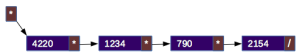In the picture above, we are inserting a node with the value 4220 at the head. In the other previous picture, we saw that the head was pointing to the node with the value 1234. So, it is necessary to copy the pointer of the head to the pointer of the next node in the new node with the value 4220 and change the head to point to this new node.

Let’s see how can we do it using C++. Notice that, in the code, we don’t know if the list is still empty! So, it is necessary to check this condition:

```    node = (Node*) malloc(sizeof(Node));
node->value=4220;
node->next = NULL;
}
else {
}
```

So, on line 3, we check if the head is NULL – meaning that the list is empty – and, in that case, the pointer of the next node shall be NULL, because it will be the first and the last node (line 4). If the list is not empty, we copy the pointer of the head to the next node (line 7). Finally, the head will now point to the new node (line 9).

### Inserting at the tail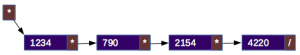In this case, we can see in the picture above, the new node with the value 4220 is inserted at the tail (end) of the list. To do so, it is necessary to access the last node to change its pointer to the next node from NULL to the new node. Let’s see how our code in C++ will look like:

```    node = (Node*) malloc(sizeof(Node));
node->value=4220;
node->next = NULL;
}
else {
while (search_last->next!=NULL) {
search_last = search_last->next;
}
search_last->next = node;
}
```

Notice that, once again, on line 4 we test if the list is empty! It it is, the insertion is equal to the insertion at the head of an empty list (line 5). Otherwise, we will have to iterate all nodes in the list, from the head to the tail (which pointer to the next node is NULL – line 9). Finally, we add the new node to the pointer “next” of the last node (line 12). Also notice that the new node has its “next” pointer set to NULL, because it will be the new last node.

We can simplify the access to the last node if we have another pointer to the tail (as we have in doubly linked lists). I’m not going to do that, but it might be a nice exercise for the reader!

### Inserting at the middle of the list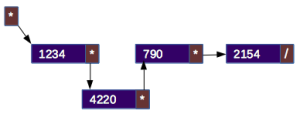Now, let’s imagine we want to insert the new node between the first and the second node. In this case, we need to access the node that precedes the position where we want to insert the new node and copy the pointer “next” of that node (in the example, the node with the value 1234) to the pointer “next” of the new node. This way, the node “1234”, which was pointing to the node with the value “790”, will now point to the new node “4220” and this one will be pointing to the node “790”. This implementation is valid for any position (after the head), including the tail. Notice that it is very similar and if we are at the tail, by copying the pointer “next” of the last node to the new node, we are copying the NULL pointer instead of a pointer to an existing next node.

By now, I’m not going to show the code for this case, because it will be used in a function to insert values in a ordered list in the third part of this article. But as a suggestion, I invite the reader to try to write this code!

In the second part we will see how to remove nodes and I’ll give you a complete example that will insert new nodes at the head and at the tail, list the values of the nodes and removes a node with a given value.

In the third and final part of this article we will see an example of implementing a list with classes.